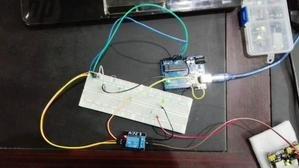# light sensor and street light control using Arduino

Light sensor and street light control using Arduino is designed to measure the intensity of light or amount of light. Street light is controlled automatically with the help of the intensity of light and Arduino. Arduino UNO R3 is used in this project. Light-dependent resistor is used for the detection of light. The relay is used to provide isolation between Arduino and 220 volt AC street light. I will explain the functionality and working of each component in later part of this article.#### Circuit diagram description :

Circuit diagram of light sensor and street light using Arduino is shown below. Now I will explain each component use in this project.

##### Light dependent resistor (LDR) :

Light dependent resistor is used to detect change in light intensity or as a light sensor. LDR is basically a variable resistor. LDR resistance changes with the change in intensity of light. If intensity of light falling on LDR is high, LDR will have low resistance. When intensity of light decreases, LDR offer high resistance. Hence there is a inverse relationship between intensity of light and resistance of LDR.

So LDR is used as a light sensor. Now the question comes to mind, how to measure resistance which in return can be used to calculate the intensity of light. As you know Arduino UNO R3 board have six analogs to digital converter channels. All analog to digital converters can measure voltage only. These channels can not measure resistance directly. But resistance can be measured indirectly by converting it into voltage form. This is basically called signal conditioning. 10K ohm resistor is used in series with LDR through a 5-volt source. This circuit is used to convert resistance into voltage form. The voltage measured across LDR can be measured with the help of analog to digital converter of Arduino. This measured voltage can be converted back into resistance using voltage division formula. I assume that you know how to measure analog voltage using analog to digital converter of Arduino UNO R3. If you don’t know how to do this. I recommend you to go through the following tutorial first before going further:

##### Relay :

In this project, a relay is used to provide isolation between low voltage circuitry and high voltage circuitry. Arduino is also used to provide a control signal to relay whenever the intensity of light falls below a certain level. The control signal is generated from pin 13 of Arduino which is used as an output pin. Transistor is used as a switch here. If you don’t know how to use input or output pins of Arduino UNO R3, read the following article :

###### “How to use input or output pins of Arduino UNO R3“

circuit diagram of light sensor and street light control using Arduino is shown below :

#### Code :

Code for light sensor and street light control using Arduino is given below :

```int Adc_channel = A0; // select the input pin for the potentiometer
int output_pin = 13; // select the pin for the LED
int light_value = 0; // variable to store the value coming from the sensor

void setup()
{
// declare the ledPin as an OUTPUT:
pinMode(output_pin, OUTPUT);
}

void loop() {
// read the value from the sensor:
// turn the ledPin on
light_value = 100 - light_value/10.24;
if(light_value>=90) // SWITCH of the light when light is 90 percent
{
digitalWrite(output_pin, LOW);
}
else
{
digitalWrite(output_pin, HIGH);
}

delay(500);

}```

The code is self-explanatory and I have also used comments with code line. But if you still have any issue while making this project, you are welcome to comment on this post. Thanks for reading this article. If you consider it helpful kindly share it with your friends.

### 16 thoughts on “light sensor and street light control using Arduino”

1. how to control the intensity of light?

2. how to control intensity

• what about 100 in code

3. your code is not compiling …

• what are you are getting?

4. what the device name of the series?

• which device?

6. i wann this circuit interfacing using 8051 microcontroller

7. Arduino: 1.6.8 (Windows 7), Board: “Arduino/Genuino Uno”

sketch_mar26b:15: error: stray ‘\342’ in program

light_value = 100 â€“ light_value/10.24;

^

sketch_mar26b:15: error: stray ‘\200’ in program

sketch_mar26b:15: error: stray ‘\223’ in program

I:\ALL btech chandana\third year sem-2\minip\implementation\four\sketch_mar26b\sketch_mar26b.ino: In function ‘void loop()’:

sketch_mar26b:15: error: expected ‘;’ before ‘light_value’

light_value = 100 â€“ light_value/10.24;

^

exit status 1
stray ‘\342’ in program

8. iam getting above error

9. exit status 1
stray ‘\226’ in program

10. there is an error in the code and it says..
“exit status 1
stray ‘\226’ in program”

11. Hello I am designing a smart street light but I am having problem over-riding the LDR
Sequenc of operation-
-3 Leds are wired to light together.
-The Leds should light only when the ambient light level is > 600.
-If a movement sensor detects movement, the Leds should light at full brightness during the period that movement is detected.
-After no further movement is detected, the Leds should remain at full brightness for 3 seconds then dim (be on half brightness ? ) for 2 seconds then switch fully off.